• 项目中遇到给出几个间隔时间点的数据，然后判断其他时刻的数据，需要整体考虑数据的变化趋势，不能通过插值来得到中间未知时刻的数据，所以需要使用多项式拟合来讲数据补全。 多项式函数是一个很重要的建模手段，...
介绍
项目中遇到给出几个间隔时间点的数据，然后判断其他时刻的数据，需要整体考虑数据的变化趋势，不能通过插值来得到中间未知时刻的数据，所以需要使用多项式拟合来将数据补全。
多项式函数是一个很重要的建模手段，利用任意个点，就可以拟合出一个多项式函数，通过多项式函数来推导出其他点的函数值，然后绘制出函数曲线，这个是最基本的原理！
拟合方法

通过点来拟合，得到拟合多项式的函数关系；
将得到的集合关系转化成多项式函数的表达式，形如xxx + axx + b*x +c 的样子；
将多项式函数表达式，解析成一个计算方法；
通过计算方法，来得到任意x（横坐标）对应的y（纵坐标）的值。

下面是用Java语言来实现多项式拟合的代码：
Java实现
拟合功能在下面代码中，已完整实现，可直接使用。
坐标实体类
定义坐标点的类，x（横坐标），y（纵坐标）
package cn.com.em.pu.fitting;

import java.io.Serializable;
import java.util.Objects;

/**
* 坐标点
*
* @author pupengfei
* @version 1.0
* @date 2020/8/27 14:45
*/
public class Point implements Serializable {

private static final long serialVersionUID = 3256087124347421878L;

private double x;

private double y;

public Point() {
}

public Point(double x, double y) {
this.x = x;
this.y = y;
}

public double getX() {
return x;
}

public void setX(double x) {
this.x = x;
}

public double getY() {
return y;
}

public void setY(double y) {
this.y = y;
}

@Override
public boolean equals(Object o) {
if (this == o) return true;
if (o == null || getClass() != o.getClass()) return false;
Point point = (Point) o;
return Double.compare(point.x, x) == 0 &&
Double.compare(point.y, y) == 0;
}

@Override
public int hashCode() {
return Objects.hash(x, y);
}

@Override
public String toString() {
return "Point{" +
"x=" + x +
", y=" + y +
'}';
}
}


自定义异常
package cn.com.em.pu.fitting;

/**
* 多项式拟合异常
*
* @author pupengfei
* @version 1.0
* @date 2020/8/27 15:29
*/
public class PolynomialFittingException extends Exception {

public PolynomialFittingException() {
}

public PolynomialFittingException(String message) {
super(message);
}

public PolynomialFittingException(String message, Throwable cause) {
super(message, cause);
}
}


多项式计算公式接口
多项式计算公式，只有一个方法，根据x获取y的值
package cn.com.em.pu.fitting;

/**
* 多项式计算公式，根据x获取y的值
*
* @author pupengfei
* @version 1.0
* @date 2020/8/27 13:57
*/
@FunctionalInterface
public interface PolynomialFnc {

/**
* 根据横坐标x，计算纵坐标y的值
* @param x 横坐标
* @return y，纵坐标
*/
double getY(double x);

}


多项式拟合工具类
数据多项式拟合工具类
package cn.com.em.pu.fitting;

import java.util.ArrayList;
import java.util.List;

/**
* 数据多项式拟合工具类
*
* @author pupengfei
* @version 1.0
* @date 2020/8/27 13:56
*/
public class PolynomialUtil {

/**
* 多项式拟合
* @param data 坐标点集合
* @return 多项式计算公式
*/
public static PolynomialFnc fitting(List<Point> data) throws PolynomialFittingException {
// 多项式每一项的计算表达式字符串
List<String> returnResult = new ArrayList<>();

int n = data.size();

List<List<Double>> inputMatrix = new ArrayList<>();

for (int i = 0; i < n; i++) {
List<Double> tempArr = new ArrayList<>();
for (int j = 0; j < n; j++) {
tempArr.add(Math.pow(data.get(i).getX(), n - j - 1));
}

tempArr.add(data.get(i).getY());
inputMatrix.add(tempArr);
}

for (int i = 0; i < n; i++) {
double base = inputMatrix.get(i).get(i);
for (int j = 0; j < n + 1; j++) {
if (base == 0) {
//存在相同x不同y的点，无法使用多项式进行拟合
throw new PolynomialFittingException("存在相同x不同y的点，无法使用多项式进行拟合");
}
inputMatrix.get(i).set(j, inputMatrix.get(i).get(j) / base);
}
for (int j = 0; j < n; j++) {
if (i != j) {
double baseInner = inputMatrix.get(j).get(i);
for (int k = 0; k < n + 1; k++) {
inputMatrix.get(j).set(k, inputMatrix.get(j).get(k) - baseInner * inputMatrix.get(i).get(k));
}
}
}
}
for (int i = 0; i < n; i++) {
if (inputMatrix.get(i).get(n) > 0) {
returnResult.add("+");
}

if (inputMatrix.get(i).get(n) != 0) {
String tmp_x = "";
for (int j = 0; j < n - 1 - i; j++) {
tmp_x = tmp_x + "*x";
}
returnResult.add((inputMatrix.get(i).get(n) + tmp_x));
}
}

// 将多项式表达式，转换为计算公式
return x -> {
double y = 0;
for (String s : returnResult) {
if ("+".equals(s)) {
y += 0;
} else if (s.contains("*")) {
String[] split = s.split("\\*");
double temp = 1;
for (String s1 : split) {
if ("x".equals(s1)) {
temp *= x;
} else {
temp *= Double.parseDouble(s1);
}
}
y += temp;
} else {
y += Double.parseDouble(s);
}
}

return y;
};
}

}


演示代码
package cn.com.em.pu.fitting;

import java.util.ArrayList;
import java.util.List;

/**
* 测试
*
* @author pupengfei
* @version 1.0
* @date 2020/8/27 20:18
*/
public class TestExample {

public static void main(String[] args) throws PolynomialFittingException {
List<Point> data = new ArrayList<>();
data.add(new Point(1, 1));
data.add(new Point(2, 2));
data.add(new Point(3, 2));
data.add(new Point(4, 1));
PolynomialFnc fitting = PolynomialUtil.fitting(data);
System.out.println(fitting.getY(3));

data = new ArrayList<>();
data.add(new Point(1, 1));
data.add(new Point(2, 3));
fitting = PolynomialUtil.fitting(data);
System.out.println(fitting.getY(3));
}

}


Jar包下载
下载地址


展开全文java 数据建模
• 本书力图从广度和深度两方面给读者以帮助，其中囊括了300多个函数的详细用法，以及图表的各种使用技巧，读者可以利月本书极大地提高工作效率。 本书话合如下读者：Excel新老版本的用户、Excel初学者、企业办公人员...
• 散点图是指在回归分析中，数据点在直角坐标系平面上分布图，散点图表示因变量随自变量而变化的大致趋势，据此可以选择合适的函数对数据点进行拟合。 　用两组数据构成多个坐标点，考察坐标点分布，判断两变量...
　　散点图是指在回归分析中，数据点在直角坐标系平面上的分布图，散点图表示因变量随自变量而变化的大致趋势，据此可以选择合适的函数对数据点进行拟合。
用两组数据构成多个坐标点，考察坐标点的分布，判断两变量之间是否存在某种关联或总结坐标点的分布模式。散点图将序列显示为一组点。值由点在图表中的位置表示。类别由图表中的不同标记表示。散点图通常用于比较跨类别的聚合数据。
散点图通常用于显示和比较数值，例如科学数据、统计数据和工程数据。

初认识：使用numpy包的random函数随机生成100组数据，然后通过scatter函数绘制散点图。

#!/usr/bin/env python
#！-*-coding:utf-8 -*-
#!@Author : Biyoulin
#!@Time   : 2018/9/2 14:40

import matplotlib.pyplot as plt
import numpy as np

plt.rcParams['font.sans-serif']=['SimHei'] #用来正常显示中文标签
plt.rcParams['axes.unicode_minus']=False   #用来正常显示负号

N = 100
x = np.random.randn(N)
y = np.random.randn(N)
plt.scatter(x,y)

plt.title("散点图示例01") #显示图表名称
plt.xlabel("x轴")    #x轴名称
plt.ylabel("y轴")    #y轴名称
plt.text(+1.2,-3,"By:biyoulin",fontsize=16,color="purple")

plt.show()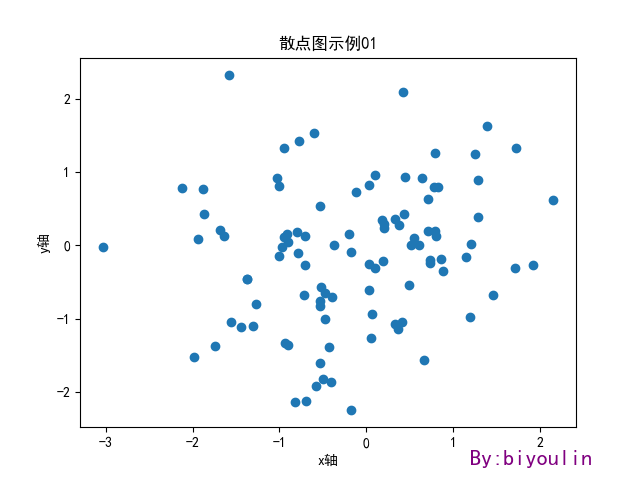scatter函数格式：

scatter(x, y, s=None, c=None, marker=None, cmap=None, norm=None, vmin=None,
vmax=None, alpha=None, linewidths=None, verts=None, edgecolors=None,
hold=None, data=None, **kwargs):

scatter函数参数详解，英文原版请参见：matplotlib官网scatter函数：

x,y：形如shape(n,)的数组，可选值，
s：  点的大小（也就是面积）默认20
c：  点的颜色或颜色序列，默认蓝色。其它如c = 'r' (red); c = 'g' (green); c = 'k' (black) ; c = 'y'(yellow)
marker：标记样式，可选值，默认是圆点；cmap: colormap，用于表示从第一个点开始到最后一个点之间颜色渐进变化；norm: normalize,vmin:vmax:alpha:  设置标记的颜色透明度，可以理解为颜色属性之一linewidths:  设置标记边框的宽度值verts:edgecolors:  设置标记边框的颜色hold:data:**kwargs:

scatter()函数各参数示例：
1、x,y：横纵坐标，数据坐标（data position）

import matplotlib.pyplot as plt
plt.scatter(x=0.5,y=0.5)
plt.show()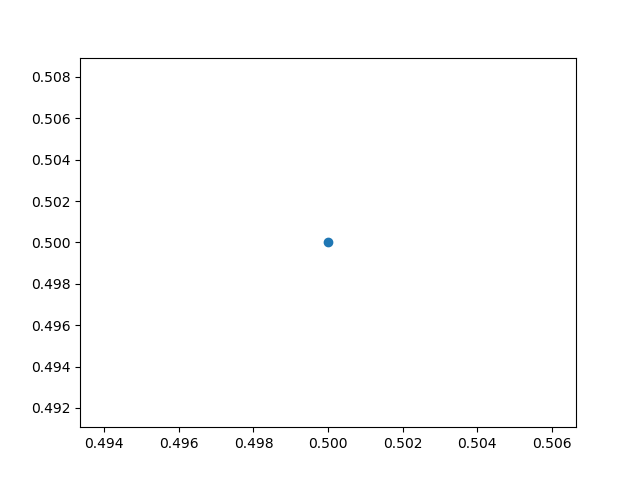2、marker : 图标，默认的是“.”,也可以是其它形状，想了解更多的，请参见：matplotlib 学习笔记02：marker标记详解。下面例子将marker设置成了“d”（diamond形状）：

import matplotlib.pyplot as plt
plt.scatter(x=0.5,y=0.5,marker='d')
plt.show()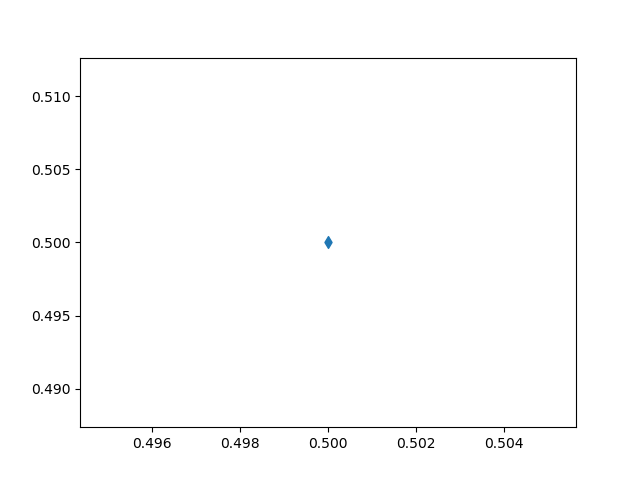也可以是文字：

import matplotlib.pyplot as plt
plt.scatter(x=0.5,y=0.5,marker='$biyoulin$')
plt.show()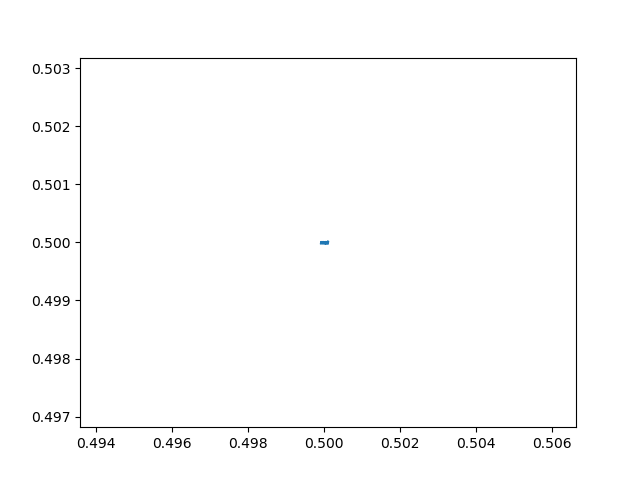上面的文字是不是看不到？因为字体太小了，通过参数s（size）可以调整“点”的大小。
3、s ： size，字体大小，默认值为20；

import matplotlib.pyplot as plt
plt.scatter(x=0.5,y=0.5,s=10000,marker='$biyoulin$')
plt.show()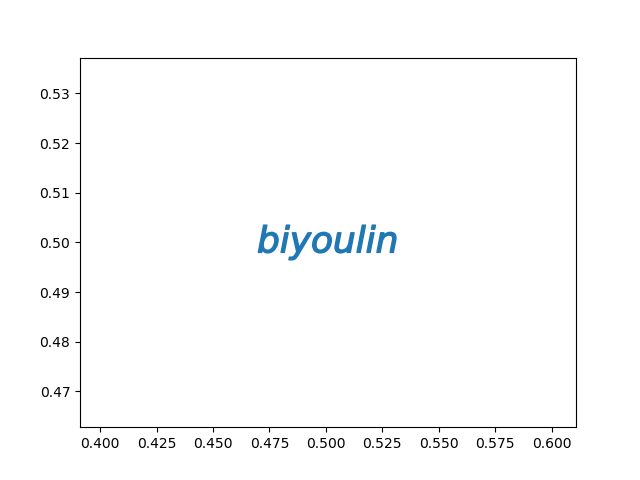到目前为止几个例子中的“点”都是蓝色的，可以通过调整c（color）参数来设置颜色：
4、c ： color，色彩会颜色序列，默认是'b'（蓝色），可支持的颜色参数如下：
b
c
g
k
m
r
w
y
blue
cyan
green
black
magenta
red
white
yellow
蓝色
青色
绿色
黑色
洋红
红色
白色
黄色

import matplotlib.pyplot as plt
plt.scatter(x=0.5,y=0.5,s=10000,c='r',marker='$biyoulin$')
plt.show()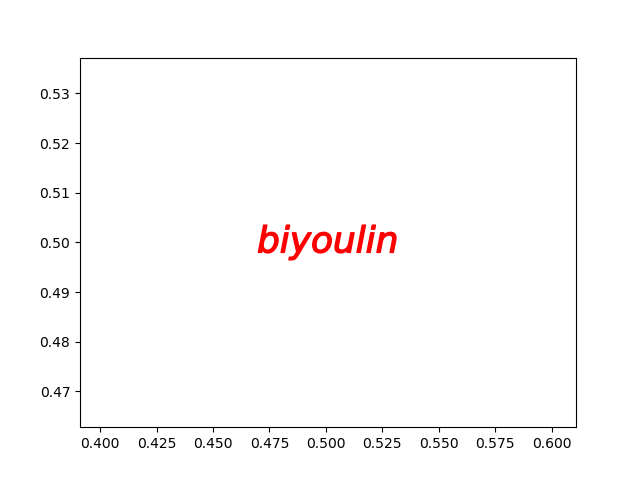5、alpha：可以理解为颜色的属性之一 ，即透明度，alpha的范围为[0,1]，从透明到不透明，上面的例子中 alpha为1，可以看看0.5的效果：

import matplotlib.pyplot as plt
plt.scatter(x=0.5,y=0.5,s=10000,c='r',alpha=0.5,marker='$biyoulin$')
plt.show()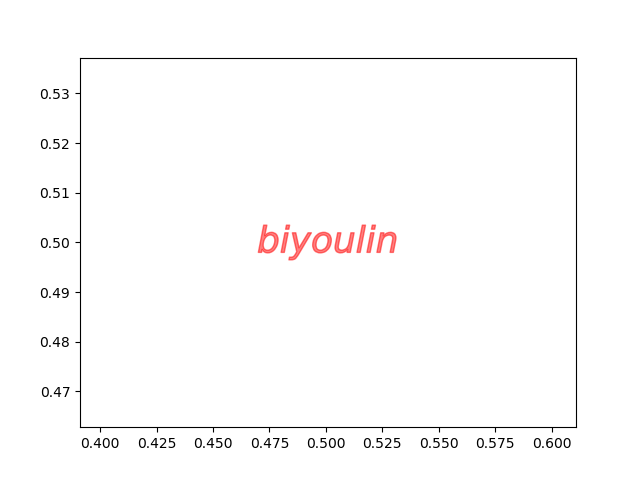6、edgecolors：the edge color of marker，顾名思义“边”的颜色，设置标记边框的颜色，下面的例子中，将边的颜色设置了为蓝色：

import matplotlib.pyplot as plt
plt.scatter(x=0.5,y=0.5,s=10000,c='r',alpha=1,marker='d',edgecolors='b')
plt.show()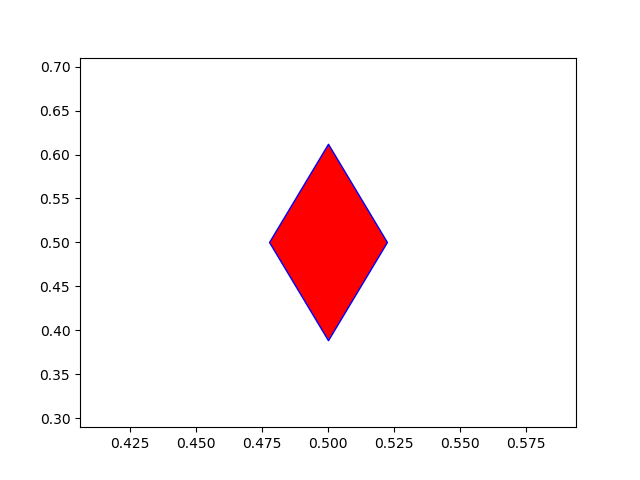可能有人会问，看不出来“边”的颜色是蓝色。没关系，通过设置linewidths，就容易看出来了。
7、linewidths：the edge size of the marker，设置标记边框的宽度；

import matplotlib.pyplot as plt
plt.scatter(x=0.5,y=0.5,s=10000,c='r',alpha=1,marker='d',linewidths=10
,edgecolors='b')
plt.show()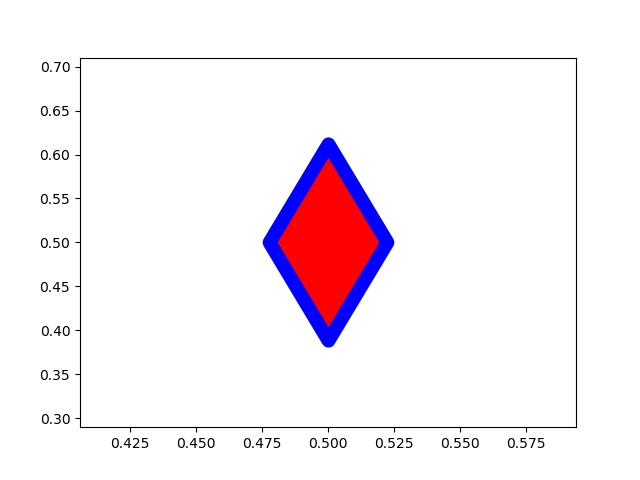8、cmap：A colormap is a series of colors in a gradient that moves from a starting to ending color。注意到是“a series of”，这个参数用于多个点之间，只有一个点就无意义了。注意到“gradient”即量级、程度，用于表示从第一个点开始到最后一个点之间颜色渐进变化。

import matplotlib.pyplot as plt
x1=list(range(0,60))
y1=list(range(0,60))
plt.scatter(x=x1,y=y1,marker='d',s=10,c=y1,cmap=plt.cm.Reds)
plt.show()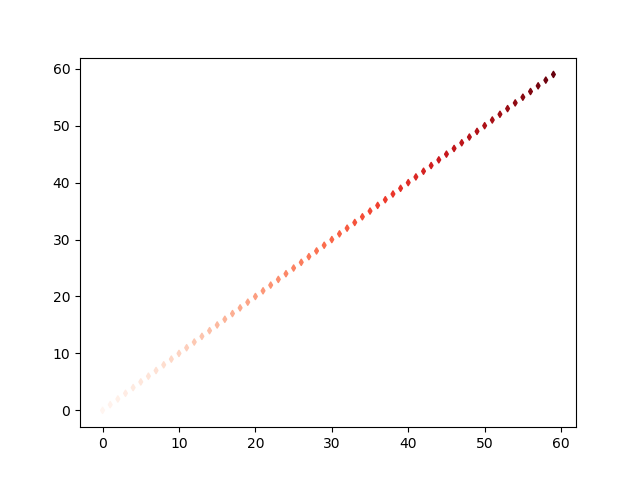注意到c=y1，不再是颜色的名称，这里是一个序列，并且值等于“点”数量值（如果不匹配，则会出错）。只有c是一个array或一个sequence，用cmap才有意义。

参考博文：https://www.cnblogs.com/sunshinewang/p/6853813.html
https://blog.csdn.net/zidephagino/article/details/80777906
作者：biyoulin
出处：http://www.cnblogs.com/biyoulin/
版权声明：本文版权归作者所有，欢迎转载，但未经作者同意必须保留此段声明，且在文章页面明显位置给出原文连接，否则保留追究法律责任的权利。
转载于:https://www.cnblogs.com/biyoulin/p/9565362.html
展开全文• 本书力图从广度和深度两方面给读者以帮助，其中囊括了300多个函数的详细用法，以及图表的各种使用技巧，读者可以利月本书极大地提高工作效率。 本书话合如下读者：Excel新老版本的用户、Excel初学者、企业办公人员...
• 本书力图从广度和深度两方面给读者以帮助，其中囊括了300多个函数的详细用法，以及图表的各种使用技巧，读者可以利月本书极大地提高工作效率。 本书话合如下读者：Excel新老版本的用户、Excel初学者、企业办公人员...
• 本书力图从广度和深度两方面给读者以帮助，其中囊括了300多个函数的详细用法，以及图表的各种使用技巧，读者可以利月本书极大地提高工作效率。 本书话合如下读者：Excel新老版本的用户、Excel初学者、企业办公人员...
• 本书力图从广度和深度两方面给读者以帮助，其中囊括了300多个函数的详细用法，以及图表的各种使用技巧，读者可以利月本书极大地提高工作效率。 本书话合如下读者：Excel新老版本的用户、Excel初学者、企业办公人员...
• 本书力图从广度和深度两方面给读者以帮助，其中囊括了300多个函数的详细用法，以及图表的各种使用技巧，读者可以利月本书极大地提高工作效率。 本书话合如下读者：Excel新老版本的用户、Excel初学者、企业办公人员...
• 本书力图从广度和深度两方面给读者以帮助，其中囊括了300多个函数的详细用法，以及图表的各种使用技巧，读者可以利月本书极大地提高工作效率。 本书话合如下读者：Excel新老版本的用户、Excel初学者、企业办公人员...
• 本书力图从广度和深度两方面给读者以帮助，其中囊括了300多个函数的详细用法，以及图表的各种使用技巧，读者可以利月本书极大地提高工作效率。 本书话合如下读者：Excel新老版本的用户、Excel初学者、企业办公人员...
• 本书力图从广度和深度两方面给读者以帮助，其中囊括了300多个函数的详细用法，以及图表的各种使用技巧，读者可以利月本书极大地提高工作效率。 本书话合如下读者：Excel新老版本的用户、Excel初学者、企业办公人员...
• 1.在判断一台master机器和一台slave是否连接通，是否能够持续连接通时候，可以使用PING命令看是否能通，后来演化成使用tcp心跳机制，每隔指定时间间隔发送心跳，最后才使用了很厉害分布式协调组件zookeeper，...
1.在判断一台master机器和一台slave是否连接通，是否能够持续连接通的时候，可以使用PING命令看是否能通，后来演化成使用tcp的心跳机制，每隔指定时间间隔发送心跳，最后才使用了很厉害的分布式协调组件zookeeper，通过创建znode，注册监听函数watcher，在znode发生变化时调用回调函数来通知master该slave机器出现了问题。

展开全文• <br>第1部分Excel入门 第1章Excel概述 第2章Excel的基本操作...判断 第10章工程函数的应用 第11章进行数学运算 第12章进行财务分析 第13章数据统计分析 第14章检测和处理信息 第15章分析...Excel
• 运用对无穷级数的一些运算组合及分析，并以Γ函数的对数微商公式作工具，深入分析了与Γ函数有关的一些特殊函数...本文的方法和结论在研究许多概率密度函数的性质时有重要作用，比如它可以判断密度曲线高度的变化趋势
• 一、算法原理 1、问题引入 ...单纯形法就解决了该问题，可以在不求导的情况下，根据若干个点的函数值大小关系，判断目标函数的变化趋势，为寻找目标函数的极值方向提供依据，这样经过若干次迭...
一、算法原理

1、问题引入

在之前讲解过的多维极值的算法中（最速下降法、牛顿法、共轭梯度法、拟牛顿法等），我们都利用了目标函数的导数值，因为函数的导数值是函数性态的反应。但在实际的工程应用中，会出现目标函数复杂导致求导麻烦甚至无法求导的情况。

单纯形法就解决了该问题，可以在不求导的情况下，根据若干个点的函数值大小关系，判断目标函数的变化趋势，为寻找目标函数的极值方向提供依据，这样经过若干次迭代，找到极值点。

例如，在求一维极值时我们用过的黄金分割法，就是根据函数值的大小关系，不断缩小搜索区间，直至满足精度，退出循环。

在求解多维极值时，我们可以使用单纯形法。

2、原理

我们以求二元函数f(x,y)极小值为例，对于二元函数需要2+1个点的函数值，构造一个三角形。

构造初始单纯性（三角形）

首先选定初始点x0=(a,b)，初始步长h0;

接着按照不同的坐标方向（x方向和y方向）和步长h0选取不同的两个点x1,x2,

x1=x0+e1*h0,x2=x0+e2*h0,式中e1，e2为步长。

以x0,x1,x2三点为顶点的三角形，即为函数f(x,y)的二维单纯形。

判断顶点处函数值

判断f(x0),f(x1),f(x2)的大小关系，将最大点记为H点，最小点记为L点，剩下的次小点即为G点。

我们要求的时f(x,y)的极小值，显然函数值越大，该点离目标点越远，反之函数值越小离目标点越近。所以我们将H点称为最坏点，L点成为最好点，G成为次好或者次坏点。

搜索方向的确定（反射）

显然，最坏点H的关于边GL的对称方向的点的函数值可能会有所改善，因此将该方向作为下一个搜索方向，这里将H点的对称点R作为下一个搜索点。

扩张和收缩

判断R点与H点的函数值大小，若R点的函数值<H点的函数值，说明该方向函数值有所下降，可以尝试让R点再走远一点(扩张)。若R点的函数值>H点的函数值，说明走的太远了，让R点收缩。

最后可以将原来的H点舍弃，选择RGL作为新的单纯形，进行上述迭代。

缩小

如果若R点的函数值>H点的函数值,收缩也不能改变函数的变化趋势，也可以将原来的单纯性HGL缩小，取GL和HL的重点C、F将CFL作为新的单纯形，进行上述迭代。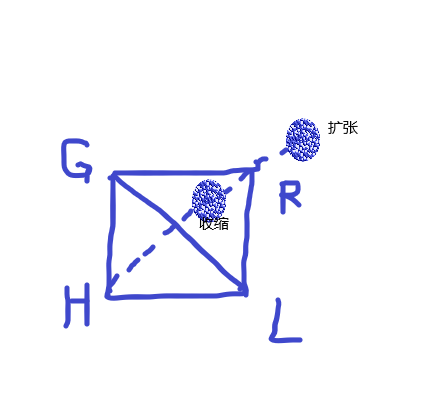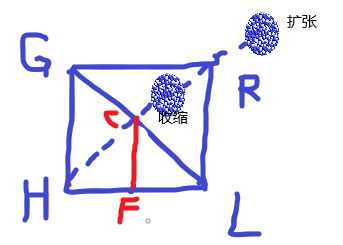matlab代码

%% 单纯形
syms x1 x2 x3 x
f=x1.^2+x2.^2-x1*x2-10*x1-4*x2+60+(x3-2)^2;
X=DCX(f,[0 0 0],1.5,1.7,0.5,1e-10,100)

function minx=DCX(f,x0,h,gama,beta,eps,k)
% f  函数符号表达式
% x0 初始点
% h  初始单纯形构造因子
% gama 扩张因子
% beta 收缩因子
% eps
% k

x0=x0';
X(:,1)=x0;%初始点x存储在顶点X的第一列
Num_var_f=length(symvar(f));%几维函数
%% 构造初始单纯形
for i = 1:Num_var_f %三维函数，需要四个顶点坐标
x = zeros(Num_var_f,1);
x(i) = x0(i) + h;
X(:,i+1)=x;% 将单纯形按列存储
end

n=1;
tol = 1 ;
while n < k && tol > eps
%% 计算函数值
for i = 1:Num_var_f+1
F_val(i)=double(subs(f,symvar(f),X(:,i)'));
end
[F_sort,F_index]=sort(F_val);%从小到大
%最好点
f_best=F_sort(1);
X_best=X(:,F_index(1));
%最差点
f_bad=F_sort(end);
X_bad=X(:,F_index(end));
%次差点
f_nextbad=F_sort(end-1);
X_nextbad=X(:,F_index(end-1));
% 计算形心
tol=abs((f_bad-f_best)/f_best);
Xc=1/Num_var_f*sum(X(:,F_index(1:end-1)),2);

%% 反射
flag = 0;
X_reflect=Xc+(Xc-X_bad);
f_reflect=double(subs(f,symvar(f),X_reflect'));
%比较反射之后
if f_best > f_reflect  % 反射点R<最好点，可以扩张
X_reflect_expand=Xc+gama*(Xc-X_bad);%扩张
f_reflect_expand=double(subs(f,symvar(f),X_reflect_expand'));
if f_reflect_expand < f_reflect %扩张点继续缩小
X(:,F_index(end)) = X_reflect_expand; %将其作为单纯性的新顶点
else %否则
X(:,F_index(end)) = X_reflect; %采用扩张前的点作为单纯形的新顶点
end
flag = 1; %反射成功标志位
end
if flag == 0  %说明没有反射成功
if f_reflect < f_nextbad  %反射点<次差点
X(:,F_index(end)) = X_reflect;%采用该反射点作为新的顶点
else  % f_reflect >= f_nextbad
if f_reflect < f_bad % 反射点要比最差点好一些
X_reflect_shrink=Xc+beta*(X_reflect-Xc);% 收缩点
f_reflect_shrink=double(subs(f,symvar(f),X_reflect_shrink'));
else
X_reflect_shrink=Xc+beta*(X_bad-Xc);% 收缩点
f_reflect_shrink=double(subs(f,symvar(f),X_reflect_shrink'));
end
if f_reflect_shrink < f_bad
X(:,F_index(end)) = X_reflect_shrink;
else % 缩短边长
X = X_best + 1/2 * (X-X_best);
end

end
end
n=n+1;
end
minx=X_best;
end



展开全文matlab 算法 动态规划 抽象代数
• 改进的稳定图利用了由输出信号的自相关函数重构的Hankel矩阵，认为系统阶次固定，模态特征的变化趋势随数据量的增加而体现。在稳定图中引入了可表征各阶估计模态贡献量大小的分量能量指标(CEI)作为判断模态特征稳定...
• 一般放在函数的开始（条件判断）。 以下问题只是为了递归练习，有些问题可能用循环解决更方便。 简单递归问题的实现： 一、用递归求阶乘 找重复：求n的阶乘就是求n*(n-1)! ，（n-1）的阶乘就是问题的重复（子问题） ...算法 数据结构 java
• 针对制造车间重调度触发机制问题, 建立了制造车间重调度损益函数, 揭示了生产车间重调度过程损失及增益的变化规律. 引入云理论测度重调度损益的不确定性, 使用逆向云算法计算重调度增益云和损失云的数字特征, 根据云...
• 3.闭包：用来解决全局污染，用来保护变量。 里面的函数使用了外面函数定义局部...循环变量：循环中做判断的量，循环变量一定是向着循环退出的趋势变化 3.循环体：循环做什么事 while(条件){干什么事...
• CPU 利用率变化量定义场景信息，用以反映相邻检查点场景信息关系，然后利用径向基函数（RBF,radial basis function）神经网络分类器评估当前检查点状态来判断软件可信情况，并运用半加权马尔可夫模型预测下一...软件可信性 检查点
• 散点图以某个特征为横坐标，以另一个特征为纵坐标，通过散点图疏密程度和变化趋势表示两个特征数量关系。常应用于显示若干数据系列中各数值之间关系，类似于x、y轴判断两变量之间是否存在某种关联。 pyplot...python 数据分析 数据可视化 matplotlib
• 如果想很快查到函数的极值或看出其发展趋势，给出的数据点也不一定非得是等差的，可以根据需要任意给定。从简单的三角函数到复杂的对数、指数函数，都可以用EXCEL画出曲线。如果用得到，你还可以利用EXCEL来完成行列...excel
• 文章目录梯度下降和方向传播1、 什么是...是一个向量，导数+变化最快的方向(学习的前进方向)即多元函数参数的变化趋势。 机器学习 收集数据x，构建机器学习模型f，得到 f(x, w) = 预测值 判断模型好坏的方法： los深度学习 人工智能 python 随机梯度下降 机器学习
• 数据库性能变化趋势判断服务器资源是否足够 selectbenchmark(100000000,'callmysp()'); #第一个函数调用多少次，第二个函数执行sql。 注意响应时间 selectbenchmark(100000000,‘select1+1')...
• 尽我所知，除了文中特别加以标注和致谢地方外，论文中不包含其他人已经发表或撰写过研究成果，也不包含为获得东南大学或其它教育机构学位或证书而使用过材料。与我一同工作同志对本研究所做任何贡献均已...
• 在顶板活动周期内,研究认为“砌体梁”结构中关键块A触矸是判断循环末阻力变化趋势的关键节点,关键块A触矸前循环末阻力逐渐增加,触矸后逐渐减小。在采煤循环周期内,研究认为“砌体梁”结构主要由煤壁、液压支架和采...
• 04075引用输入了某个函数的全部单元格 04076引用含有特定文本字符串的所有单元格 04077引用设置了数据有效性的所有单元格 04078获取单元格地址 04079获取单元格区域内的单元格数 04080获取单元格区域的行数 04081...Excel VBA
• 散点图（scatter diagram）表示因变量随自变量而变化的大致趋势，据此可以选择合适的函数对数据点进行拟合。用两组数据构成多个坐标点，考察坐标点分布，判断两变量之间是否存在某种关联或总结坐标点分布模式。...